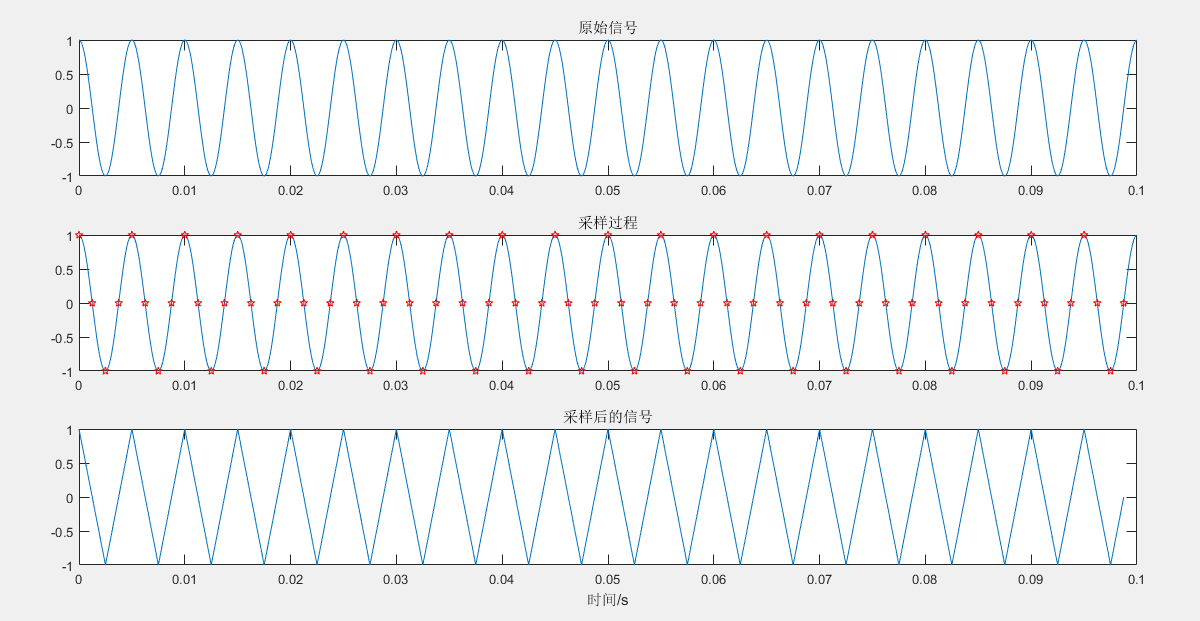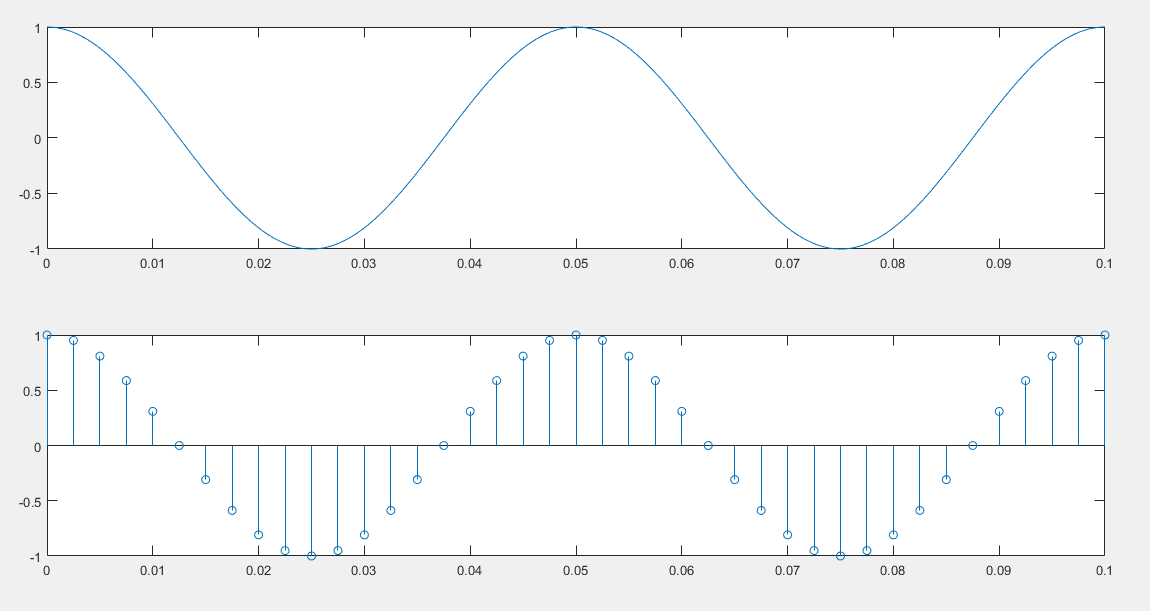# MATLAB信号处理之信号的采样

f=200;               %信号频率为200Hz
t=(0:0.0001:0.1);    %定义信号的时间范围
x=cos(2*pi*f*t);     %生成信号

fs=800;              %采样频率为800Hz
N=80;                %定义采样点数
dt=1/fs;             %采样间隔，采样间隔其实就可以理解为是采样信号的周期，周期=1/频率
T=(0:N-1)*dt;        %定义采样的每个时间点
x1=cos(2*pi*f*T);    %对信号进行采样

subplot(311);
plot(t,x);
ylim([-1 1])
title('原始信号')
subplot(312)
plot(t,x,T,x1,'rp');
ylim([-1 1]);
title('采样过程')

subplot(313)
plot(T,x1);
ylim([-1 1])
xlabel('时间/s')
title('采样后的信号')fs=800;           %采样频率为800Hz
dt=1/fs;          %采样间隔，采样间隔其实就可以理解为是采样信号的周期，周期=1/频率
T=0:dt:0.1;       %定义采样的每个时间点
x1=cos(2*pi*f*T); %对信号进行采样

f=20;                  %信号频率为20Hz
t=(0:0.0001:0.1);      %定义信号的时间范围
x=cos(2*pi*f*t);       %生成信号

fs=400;                %采样频率为400Hz
dt=1/fs;               %采样间隔，采样间隔其实就可以理解为是采样信号的周期，周期=1/频率
T=0:dt:0.1;            %定义采样的每个时间点
x1=cos(2*pi*f*T);      %对信号进行采样

subplot(211)
plot(t,x)
subplot(212)
stem(T,x1)